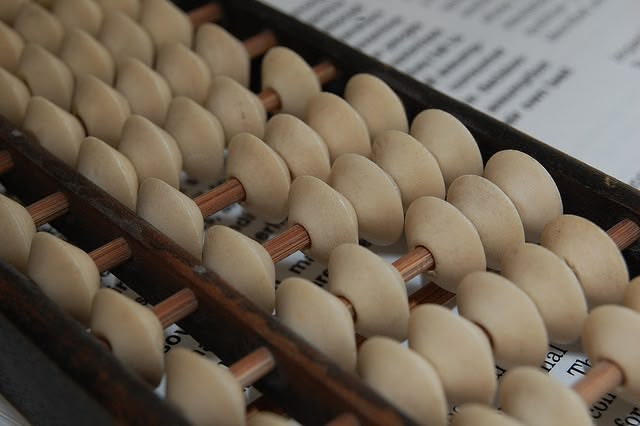A mental math installment:

While walking to Carnegie Mellon the other day I started thinking about squaring two digit numbers. So instead of hoarding my findings and short amount of research I thought I would write a tutorial on how to square two digit numbers, and then some.

Method 1: If You Know the Previous Square

This method is only marginally helpful, but will come in handy if you know how to easily square the number previous to the number you’re trying to square. Let’s say you’re trying to find x2. If you know what (x-1)2 is already, all you have to do is add x and (x-1) to (x-1)2 to find x2.

Proof:

(x-1)2 + x + (x-1) = (x2 - 2x + 1) + x + x - 1 =
(x2 - 2x + 1) + 2x - 1 =
x2 + (2x - 2x) + (1 - 1) =
x2

q.e.d.

Example:

Let’s find 312 knowing that 302 = 900.

312 = 302 + 31 + 30 = 900 + 31 + 30 = 961.

This method is mostly helpful for squaring numbers which are one more than a multiple of ten, since humans can square multiples of ten without much thought (more on this later). Also, you’re not restricted to squaring two digit numbers with this method, which is quite fantastic.

Method 2: Multiplying One Up, One Down, and then Adding One

In my opinion this method is a bit more fun, but it definitely requires some mental multiplication. In fact, you’ll almost be doing as much work (or more) using this method as you would be in outrightly squaring a number, but it is quite an amusing trick.

Let’s say you want to square a number x. If you multiply (x-1) and (x+1) together, and then add 1, you’ll find x2.

Proof:

(x-1)(x+1) + 1 =
(x2 + x - x - 1) + 1 =
x2 + (x - x) + (1 - 1) =
x2

q.e.d.

Example:

Let’s find 312 again using this trick.

312 = (30)(32) + 1 = 960 + 1 = 961.

Like I said, if you can’t quickly calculate that (30)(32) is 960, then this method isn’t saving you much mental energy, but this averaging method could be a shortcut for some.

Method 3: Squaring Two Easy Numbers Instead of One Hard Number (Plus a Step)

In my opinion, this method is best practice. This explanation will be a bit lengthier, and also a bit harder to write out in plain English, but it’s the most fruitful by far of the three methods.

Like I said in my previous post on mental math, perhaps the most extensive repository most folks have for math is their times tables. With some exception, people are able by their mid-teens to multiply together two one digit numbers with relative ease, all the way up to (9)(9) = 81. Let’s make use of this fact, plus the fact that folks can easily multiply multiples of ten, to square any two digit number easily.

First, one needs to realize that any two digit number is at most five numbers away from a multiple of ten. For instance, 34 is 4 numbers away from 30, and 65 is 5 numbers away from both 60 and 70. So when we’re thinking of squaring large two digit numbers it’s best to think of it as a multiple of ten (e.g. 10, 20, 30, 40, …) plus or minus a number no greater than five.

So when we go to square a number like 74 mentally (yikes!), it’s better for one to imagine this number not as 74 but as (70 + 4), or to imagine the number 66 not as a single number, but as the difference between two, i.e. (70 - 4). It’s a matter of breaking down a difficult single process into several easier ones.

So let’s find 742. Instead of looking at the single number 74, let’s break it down into (70 + 4). Now let’s square this number.

(70 + 4)2 =
(70 + 4)(70 + 4)

At this point in the game we’re multiplying two binomials together. Remember the FOIL (Front, Outer, Inner, Last) method from way back when? I thought so. Continuing with this method we have

702 + (70)(4) + (4)(70) + 42
4900 + 280 + 280 + 16 (a)

We’ve broken the process down into more or less multiplying single digit numbers together and then adding zeroes at the end. We know 72 = 49, (7)(4) = (4)(7) = 28, and 42 = 16, and it’s then easy to multiply these numbers by powers of ten.

Adding these numbers up we get 5476. We’ve taken a difficult multiplication problem and turned it into an easier addition problem (though some would beg to differ, I’m sure). It works the same for a number like 66, which looks like (70 - 4) when we break it down. The only difference is that we’ve traded our plus sign for a negative one. The only part of the arithmetic that changes is that instead of adding 280 twice to expression (a) above, we subtract it twice. So similarly to 742 we have that

662 =
4900 - 280 - 280 + 16 =
4356

And this method, in my opinion, is by far the easiest way to mentally square two digit numbers. A similar process will work with three digit numbers. Perhaps I’ll write on that later.

There you go. Hopefully the next time you need to square a number quickly you’ll be more equipped for usual. So for now, happy squaring, and let me know if anyone has any suggestions or additions.

Note: For all you nitpickers out there let it be known that when I’ve used the word “number” at any point in this post I’ve actually meant this word to mean “positive integer”.